Courses

# Ex 5.1 NCERT Solutions- Arithmetic Progressions Class 10 Notes | EduRev

## Class 10 : Ex 5.1 NCERT Solutions- Arithmetic Progressions Class 10 Notes | EduRev

The document Ex 5.1 NCERT Solutions- Arithmetic Progressions Class 10 Notes | EduRev is a part of the Class 10 Course Mathematics (Maths) Class 10.
All you need of Class 10 at this link: Class 10

Ques 1: In which of the following situations, does the list of numbers involved make an arithmetic progression, and why?
(i) The taxi fare after each km when the fare is ₹ 15 for the first km and ₹ 8 for each additional km.
(ii) The amount of air present in a cylinder when a vacuum pump removes 1/4 of the air remaining in the cylinder at a time.
(iii) The cost of digging a well after every metre of digging, when it costs ₹ 150 for the first metre and rises by ₹ 50 for each subsequent metre.
(iv) The amount of money in the account every year, when ₹ 10,000 is deposited at compound interest at 8% per annum.
Sol: (i) Let us consider,
The first term (T1) = Fare for the first km = ₹ 15 since, the taxi fare beyond the first km is ₹ 8 for each additional km. ⇒ T1 = 15
∴ Fare for 2 km = ₹15 + 1 × ₹ 8 ⇒ T2 = a + 8 [where a = 15]
Fare for 3 km = ₹ 15 + 2 × ₹ 8 ⇒ T3 = a + 16
Fare for 4 km = ₹ 15 + 3 × ₹ 8 ⇒ T4 = a + 24
Fare for 5 km = ₹ 15 + 4 × ₹ 8 ⇒ T5 = a + 32
Fare for n km = ₹ 15 + (n - 1) 8 ⇒ Tn = a + (n - 1) 8
We see that above terms form an A.P.
(ii) Let the amount of air in the cylinder = x
∴ Air removed in 1st stroke =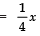⇒ Air left after 1st stroke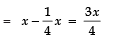Air left after 2nd stroke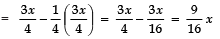Air left after 3rd stroke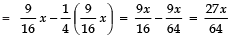Air left after 4th stroke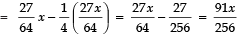Thus, the terms are: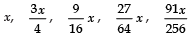Here,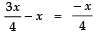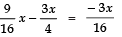Since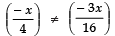The above terms are not in A.P.
(iii) Here, The cost of digging for 1st metre = ₹ 150
The cost of digging for first 2 metres = ₹ 150 + ₹ 50 = ₹ 200
The cost of digging for first 3 metres = ₹ 150 + (₹ 50) × 2 = ₹ 250
The cost of digging for first 4 metres = ₹ 150 + (₹ 50) × 3 = ₹ 300
∴ The terms are: 150, 200, 250, 300, ...
Since, 200 - 150 = 50
And 250 - 200 = 50
⇒ (200 - 150) = (250 - 200)
∴The above terms form an A.P.
(iv) ∵ The amount at the end of 1st year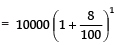The amount at the end of 2nd year =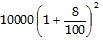The amount at the end of 3rd year =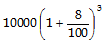The amount at the end of 4th year =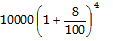∴ The terms are
Obviously,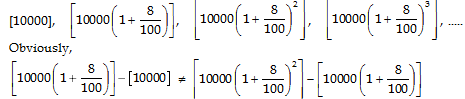∴ The above terms are not in A.P.

Ques 2: Write first four terms of the AP, when the first term a and the common difference d are given as follows:
(i) a = 10, d = 10
(ii) a = - 2, d = 0
(iii) a = 4, d = - 3
(iv) a = - 1, d = 0
(v) a = - 1.25, d = - 0.25
Sol: (i) ∵ Tn = a + (n - 1) d
∴ For a = 10 and d = 10, we have:
T1 = 10 + (1 - 1) × 10 = 10 + 0 = 10
T2 = 10 + (2 - 1) × 10 = 10 + 10 = 20
T3 = 10 + (3 - 1) × 10 = 10 + 20 = 30
T4 = 10 + (4 - 1) × 10 = 10 + 30 = 40
Thus, the first four terms of A.P. are:
10, 20, 30, 40.
(ii) ∵ Tn = a + (n - 1) d
∴ For a = - 2 and d = 0, we have:
T1 = -2 + (1 - 1) × 0 = -2 + 0 = -2
T2 = -2 + (2 - 1) × 0 = -2 + 0 = -2
T3 = -2 + (3 - 1) × 0 = -2 + 0 = -2
T4 = -2 + (4 - 1) × 0 = -2 + 0 = -2
∴ The first four terms are:
- 2, - 2, - 2, - 2.
(iii) ∵ Tn = a + (n - 1) d
∴ For a = 4 and d = - 3, we have:
T1 = 4 + (1 - 1) × (- 3) = 4 + 0 = 4
T2 = 4 + (2 - 1) × (- 3) = 4 + (- 3) = 1
T3 = 4 + (3 - 1) × (- 3) = 4 + (- 6) = - 2
T4 = 4 + (4 - 1) × (- 3) = 4 + (- 9) = - 5
Thus, the first four terms are:
4, 1, - 2, - 5.
(iv) ∵ Tn = a + (n - 1) d
For a = - 1 and d = 1/2, we get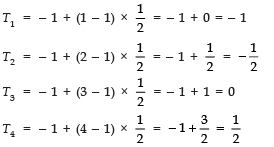∴ The first four terms are: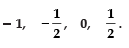(v) ∵ Tn = a + (n - 1) d
For a = - 1.25 and d = - 0.25, we get
T1 = - 1.25 + (1 - 1) × (- 0.25) = - 1.25 + 0 = - 1.25
T2 = - 1.25 + (2 - 1) × (- 0.25) = - 1.25 + (- 0.25) = - 1.50
T3 = - 1.25 + (3 - 1) × (- 0.25) = - 1.25 + (- 0.50) = - 1.75
T= - 1.25 + (4 - 1) × (- 0.25) = - 1.25 + (- 0.75) = - 2.0
Thus, the four terms are:
- 1.25, - 1.50, - 1.75, - 2.0

Ques 3: For the following APs, write the first term and the common difference:
(i) 3, 1, - 1, - 3, ...
(ii) - 5, - 1, 3, 7, ...
(iii)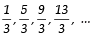...
(iv) 0.6, 1.7, 2.8, 3.9, ...
Sol: (i) We have: 3, 1, - 1, - 3, .....
⇒ T1 = 3 ⇒ a = 3
T2 = 1
T3 = - 1
T4 = - 3
∴ T2 - T1 = 1 - 3 = - 2
T4 - T3 = - 3 - (- 1) = - 3 + 2 = - 2 ⇒ d = - 2
Thus, a = 3 and d = - 2
(ii) We have: - 5, - 1, 3, 7, .....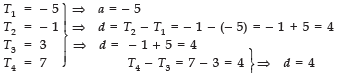Thus, a = -5 and d = 4
(iii) We have: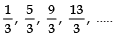.....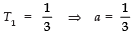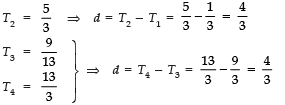Thus, a =1/3 and d = 4/3
(iv) We have: 0.6, 1.7, 2.8, 3.9, .....
⇒ T1 = 0.6⇒ a = 0.6
T2 = 1.7 ⇒ d = T2 - T1 = 1.7 - 0.6 = 1.1
T3 = 2.8
T4 = 3.9 ⇒ d = T4 - T3 = 3.9 - 2.8 = 1.1
Thus, a = 0.6 and d = 1.1

Ques 4: Which of the following are APs? If they form an AP, find the common difference d and write three more terms.
(i) 2, 4, 8, 16, ...
(ii)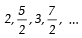...
(iii) - 1.2, - 3.2, - 5.2, - 7.2, ...
(iv) - 10, - 6, - 2, 2, ...
(v)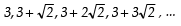(vi) 0.2, 0.22, 0.222, 0.2222, ...
(vii) 0, - 4, - 8, - 12, ...
(viii)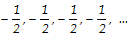(ix) 1, 3, 9, 27, ...
(x) a, 2a, 3a, 4a, ...
(xi) a, a2, a3, a4, ...

(xii) √2,√8 √18 √ 32...
(xiii) √3, √6 √9, √12, ...

(xiv) 12, 32, 52, 72, ...
(xv) 12, 52, 72, 73, ...
Sol: (i) We have: 2, 4, 8, 16, .....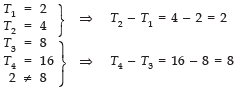∴ T2 - T1 ≠ T4 - T3
∴ The given numbers do not form an A.P.
(ii) We have: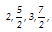, .....
∴T1 = 2, T2 = 5/2, T3 = 3, T4 = 7/2
T2 - T1 =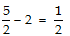T3 - T2 =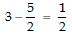T4 - T3 =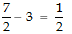∵ T2 - T1 = T3 - T2 = T4 - T3 = 1/2 ⇒ d = 1/2
∴The given numbers form an A.P.
∴T5 =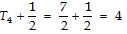T6 =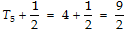T7 =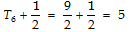Thus, d = 1/2 and T5 = 4, T6 = 9/2 and T7 = 5
(iii) We have: - 1.2, - 3.2, - 5.2, - 7.2, .....
∴T1 = -1.2, T2 = -3.2, T3 = -5.2, T4 = -7.2
T2 - T1 = -3.2 + 1.2 = -2
T3 - T2 = -5.2 + 3.2 = -2
T4 - T3 = -7.2 + 5.2 = -2
∵ T2 - T1 = T3 - T2 = T4 - T3 = -2 ⇒ d = -2
∴ The given numbers form an A.P.
Such that d = - 2.
Now, T5 = T4 + (- 2) = - 7.2 + (- 2) = - 9.2
T6 = T5 + (- 2) = - 9.2 + (- 2) = - 11.2
T7 = T6 + (- 2) = - 11.2 + (- 2) = - 13.2
Thus, d = - 2 and T5 = - 9.2, T6 = - 11.2 and T7 = - 13.2
(iv) We have: - 10, - 6, - 2, 2, .....
∴ T1 = -10, T2 = -6, T3 = -2, T4 = 2
T2 - T1 = -6 + 10 = 4
T3 - T2 = -2 + 6 = 4
T4 - T3 = 2 + 2 = 4
∵ T2 - T1 = T3 - T2 = T4 - T3 = 4 ⇒ d = 4
∴The given numbers form an A.P.
Now, T5 = T4 + 4 = 2 + 4 = 6
T6 = T5 + 4 = 6 + 4 = 10
T7 = T6 + 4 = 10 + 4 = 14
Thus, d = 4 and T5 = 6, T6 = 10, T7 = 14
(v) We have: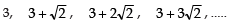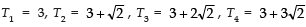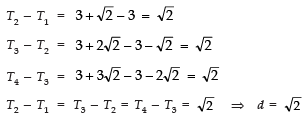⇒ The given numbers form an A.P.
Now, T5 = T4+√2
= 3+3√2+√2 = 3+4√2
T6 = T5+ √2
= 3+4√2+√2 = 3+5√2
T7 = T6+√2
= 3+5√2+√2 = 3+6√2
Thus, d = √2 and T5 = 3+4√2, T6 = 3+5√2, T7 = 3.+6√2.
(vi) We have: 0.2, 0.22, 0.222, 0.2222, .....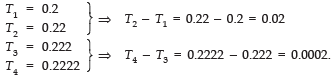Since,
T2 - T1 ≠ T4 - T3
∴ The given numbers do not form an A.P.
(vii) We have: 0, - 4, - 8, - 12, .....
∴ T1 = 0, T2 = -4, T3 = -8, T4 = -12
T2 - T1 = -4 - 0 = -4
T3 - T2 = -8 + 4 = -4
T4 - T3 = -12 + 8 = -4
∵ T2 - T1 = T3 - T2 = T4 - T3 = -4 ⇒ d = -4
∴The given numbers form an A.P.
Now, T5 = T4 + (- 4) = - 12 + (- 4) = - 16
T6 = T5 + (- 4) = - 16 + (- 4) = - 20
T7 = T6 + (- 4) = - 20 + (- 4) = - 24
Thus, d = - 4 and T5 = - 16, T6 = - 20, T7 = - 24
(viii) We have: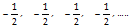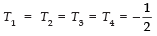T2 - T1 = 0
T3 - T2 = 0
T4 - T3 = 0
∵ T2 - T1 = T3 - T2 = T4 - T3 = 0 ⇒ d = 0
∴ The given number form an A.P.
Now,
T5 =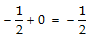T6 =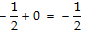T7 =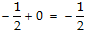Thus,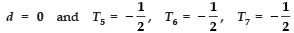(ix) We have: 1, 3, 9, 27, .....
Here,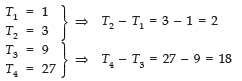∵ T2 - T1 ≠ T4 - T3
∴The given numbers do not form an A.P.
(x) We have: a, 2a, 3a, 4a, .....
∴ T1 = a, T2 = 2a, T3 = 3a, T4 = 4a
T2 - T1 = 2a - a = a
T3 - T2 = 3a - 2a = a
T4 - T3 = 4a - 3a = a
∵  T2 - T1 = T3 - T2 = T4 - T3 = a ⇒ d = a
∴The numbers form an A.P.
Now, T5 = T4 + a = 4a + a = 5a
T6 = T5 + a = 5a + a = 6a
T7 = T6 + a = 6a + a = 7a
Thus, d = a and T5 = 5a, T6 = 6a, T7 = 7a
(xi) We have: a, a2, a3, a4, .....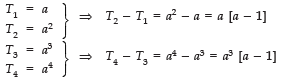Since,
T2 - T1 ≠ T4 - T3
∴ The given terms are not in A.P.
(xii) We have: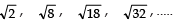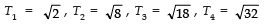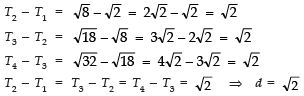∴ The given numbers form an A.P.
Now,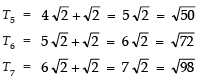Thus,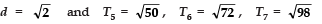(xiii) We have: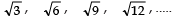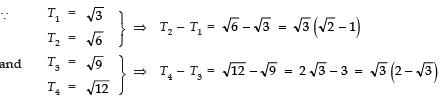∴ T2 - T1 ≠ T4 - T3
⇒ The given terms do not form an A.P.
(xiv) We have: 12, 32, 52, 72, .....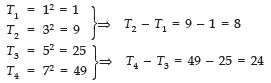∵ T2 − T1 ≠ T4 − T3
∴ The given terms do not form an A.P.
(xv) We have: 12, 52, 72, 73, .....
∴ T1 = 12 = 1, T2 = 52 = 25, T3 = 72 = 49, T4 = 73
T2 - T1 = 25 - 1 = 24
T3 - T2 = 49 - 25 = 24
T4 - T3 = 73 - 49 = 24
∵ T2 - T1 = T3 - T2 = T4 - T3 = 24 ⇒ d = 24
∴ The numbers form an A.P.
Now, T5 = T4 + 24 = 73 + 24 = 97
T6 = T5 + 24 = 97 + 24 = 121
T7 = T6 + 24 = 121 + 24 = 145
Thus, d = 24 and T= 97, T6 = 121, T7 = 145
nth Term of an A.P.

The nth term Tn of the A.P. with first term ‘a’ and common difference ‘d’ is given by
Tn = a + (n - 1) d
‘Tn’ is also called the general term of the A.P. If there are ‘m’ terms in the A.P., then ‘Tm’ represents the last term which is generally denoted by ‘l’.

Offer running on EduRev: Apply code STAYHOME200 to get INR 200 off on our premium plan EduRev Infinity!

## Mathematics (Maths) Class 10

178 videos|268 docs|103 tests

,

,

,

,

,

,

,

,

,

,

,

,

,

,

,

,

,

,

,

,

,

;